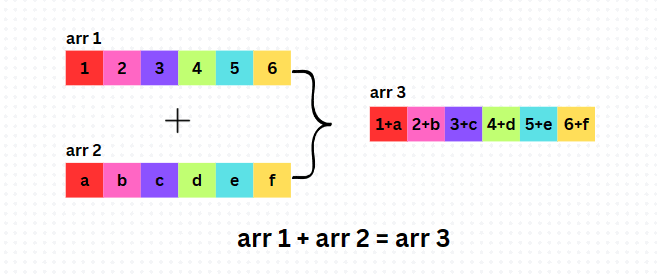### Get started with Spring 5 and Spring Boot 2, through the Learn Spring course:

>> CHECK OUT THE COURSE

## 1. Overview

Arrays are one of the most commonly used data structures in Java. They allow us to store multiple values of the same type in a single variable. Sometimes, we may need to perform some operations on the elements of two or more arrays, such as adding, subtracting, multiplying, or dividing them.

In this tutorial, we’ll focus on how to calculate the sum of two arrays, element by element, in Java.

## 2. Different Ways of Summing Arrays

Calculating the sum of arrays is a common and useful task in Java for various reasons. Some of the possible applications are:

• performing arithmetic operations on vectors or matrices
• combining or merging data from different sources or formats
• performing statistical analysis or data manipulation on numerical data etc.

To calculate the sum of two arrays, both of them must be of equal type and size. If they have different types or sizes, we’d get an IllegalArgumentException. To solve this problem, we’ve to create a third array of the same size and then store the sum of the corresponding elements of the given arrays:Let’s explore different ways to do this.

### 2.1. Using a For Loop

A for loop is the most basic and straightforward way to iterate over the elements of both arrays and add them together. We can use a for loop with an index variable that goes from 0 to the length of the arrays minus one.

Inside the loop, we can access each element of both arrays using the index variable and store their sum in the third array at the same index. Let’s calculate the sum of two arrays using this method:

``````public int[] sumOfTwoArrays(int[] arr1, int[] arr2) {
int[] arr3 = new int[arr1.length];
for (int i = 0; i < arr1.length; i++) {
arr3[i] = arr1[i] + arr2[i];
}
return arr3;
}``````

### 2.2. Using a For-each Loop

The for-each loop is a simplified version of the for loop which doesn’t require an index variable. Instead, it uses a variable that holds each element of one array and iterates over all elements.

Inside the loop, we can access each element of the other array using a counter variable that increments with each iteration. We can then store their sum in the third array at the same counter value. Let’s implement this next:

``````public int[] sumOfTwoArrays(int[] arr1, int[] arr2) {
int[] arr3 = new int[arr1.length];
int counter = 0;
for (int num1 : arr1) {
arr3[counter] = num1 + arr2[counter];
counter++;
}
return arr3;
}``````

### 2.3. Using Streams

This is a more advanced and functional way of calculating arrays in Java. Streams are sequences of data that support various operations such as filtering, mapping, reducing, etc.

We can use streams to convert both arrays into IntStream objects, streams of primitive int values. We can then use the range method from the IntStream class to create a stream of indices from 0 to the minimum length of the two arrays. Next, we can use the map method to apply a function that adds the corresponding elements of the two arrays and returns an int value. Finally, we can use the toArray method to collect the resulting stream into an int array:

``````public static int[] sumOfTwoArrays(int[] arr1, int[] arr2) {
IntStream range = IntStream.range(0, Math.min(arr1.length, arr2.length));
IntStream stream3 = range.map(i -> arr1[i] + arr2[i]);
int[] arr3 = stream3.toArray();
return arr3;
}
``````

## 3. Comparison of the Different Methods

Let’s compare and contrast the advantages and disadvantages of these methods in terms of simplicity, readability, performance, memory usage, etc.

### 3.1. Using a For Loop

The for loop is the simplest and most straightforward calculation method. It doesn’t require any special syntax or features. It is also easy to understand and debug as it follows a clear and sequential logic.

However, using a for loop can have some disadvantages. It requires an index variable to access each element of the arrays, which can introduce errors or off-by-one bugs. It also requires a third array to store the sum values, which can increase memory usage and the risk of ArrayIndexOutOfBounds exceptions. This makes it less efficient than the others.

### 3.2. Using a For-each Loop

The for-each loop is more concise and elegant. It doesn’t require an index variable. It iterates over the elements directly and uses a counter variable to access the corresponding elements of the other array. The syntax is more readable and intuitive.

However, it also requires a third array to store the sum values, which increases memory usage. Also, using a counter variable can introduce errors or off-by-one bugs if not handled carefully.

### 3.3. Using Streams

This method is more functional and expressive. It doesn’t require any index or counter variable as it uses streams to manipulate the arrays as sequences of elements. It creates a third array internally using the toArray() method.

On the downside java.util.stream can increase the verbosity and complexity of the code.

## 4. Conclusion

In this article, we’ve learned how to calculate the sum of two arrays element by element in Java.

The code examples are available over on GitHub.

### Get started with Spring 5 and Spring Boot 2, through the Learn Spring course:

>> CHECK OUT THE COURSE
1 Comment
Inline Feedbacks